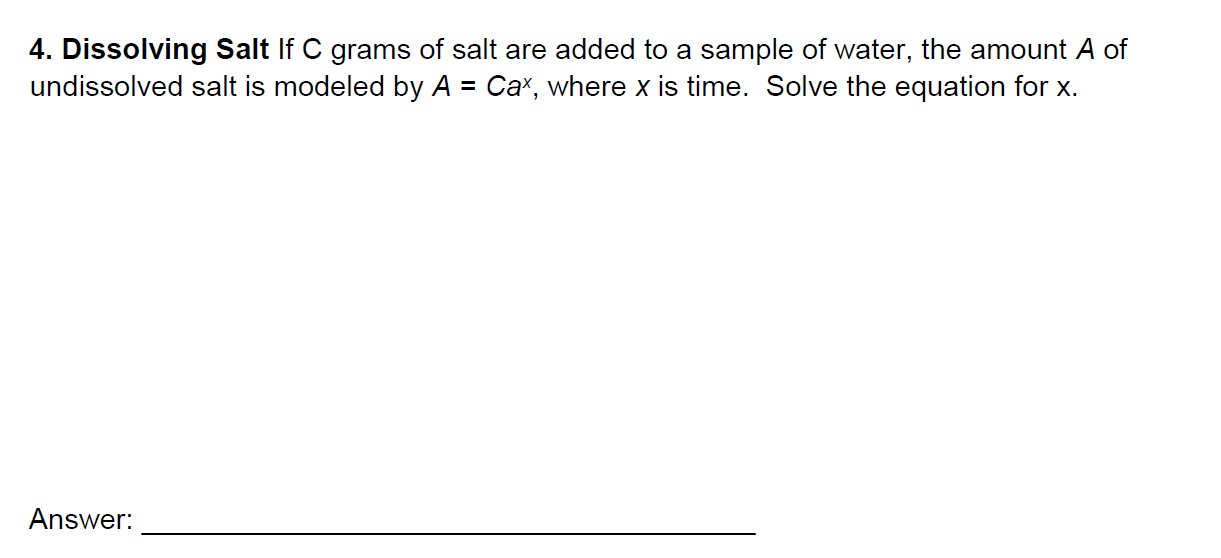4. Dissolving Salt If C grams of salt are added to a sample of water, the amount A ofundissolved salt is modeled by A Ca, where x is time. Solve the equation for x.Answer:

Question

Please answer the attached question. Thanks a lothelp_outlineImage Transcriptionclose4. Dissolving Salt If C grams of salt are added to a sample of water, the amount A of undissolved salt is modeled by A Ca, where x is time. Solve the equation for x. Answer: fullscreen
Step 1

The given equation is A = Cax.

Step 2

Simplify the expression A = Cax as follows.

Step 3

Take common logarithm ...

Want to see the full answer?

See Solution

Want to see this answer and more?

Our solutions are written by experts, many with advanced degrees, and available 24/7

See Solution
Tagged in

Other